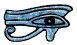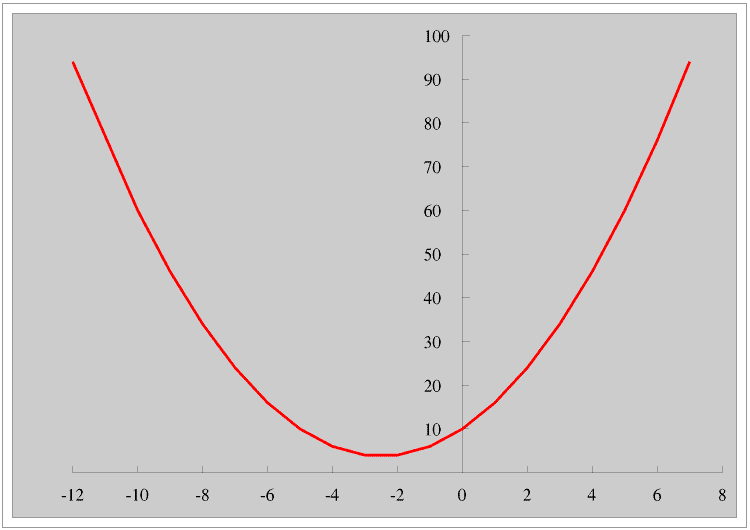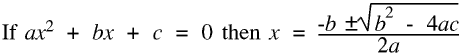## Introduction

Our word quadratic comes from the Latin word quadratus which means a square, so quadratics include squared numbers such as and . Something that contains only x and y is liniar, if it contains and it is cubic.

Here is the graph of y = + 5x +10 for -12<x<8It is a quadratic curve called a parabola. (Circles and ellipses are also quadratic curves but their graphs include as well as x².) All parabolas are a similar shape, although they can be the other way up, and have an axis of symmetry. If you throw a ball across an open space it follows a parabolic trajectory as it first gets higher and then starts to fall again.From the first graph you can see that x can have any value, and for every value of x there is only one value of y, but y has a minimum value (or a maximum in the second graph), and for every value of y above this value there are two values of x. Finding the value of y given the value of x is very simple and should take you only a few seconds even without a calculator, finding both values of x given the value of y is much more difficult.

If we are given y = + 3x + 10 and want to find the value of x when y is 6 we can write this down as + 3x + 10 = 6

First we turn it into a quadratic equation. A quadratic equation is always of the form

a + bx + c = 0

where a, b and c are constants. To do this we subtract 6 from both sides to give us the quadratic equation +3x + 4 = 0

There are three ways of solving a quadratic equation, that is, finding the value of x: factorisation, completing the square, and using the formula.

## Factorisation

If we have (x + p)(x + q) and multiply it out we have
+ qx + px + pq

or + (p + q)x +pq

So (x + 3)(x + 4) = + 7x + 12

To factorise + 5x + 6 we look for two numbers which multiplied together make 6 and added together make 5. Best to work through the factors of 6 starting with the smallest, that is 1: 6 = 1 × 6, no good, try 2 × 3 bingo!

If negative numbers are involved just remember 12 is +3 × +4 or -3 × -4, and -12 is +3 × -4 or -3 × +4

For example -2x -24 = (x + 4)(x - 6)

Once we have found the factors we can solve the equation. If (x + 2)(x - 3) = 0 then either (x + 2) = 0 or (x - 3) = 0 so x = -2 or x = +3

Factorisation is not always possible, so if you do not succeed after two or three attempts try the next method, completing the square.

If there is a number in front of the for example 6 + 19x + 15 factorisation is sometimes possible but only if you are very confident about what you are doing. Unless you are it is safer to use the formula.

## Completing the square

(x + n)² = +2n + n² so - 6x + 9 is (x - 3)²
If + 6x -11 = 0 then + 6x + 9 = 20 so (x +3)² = 20
so x + 3 = ±√(20) so x = -3 ±√(20) Don’t forget the plus or minus!

We complete the square by halving the number in front of the x and then squaring it. Then we add or subtract numbers to the right hand side of the equation to leave it balanced but with just the square on the left hand side.

Remenber that there is no (real - this is explained below) value of the square root of a negative number - look at the graph of the parabola at the top of this Page to see that for values of y below about 3 there is no real value of x.

## The Quadratic Equation

Here is the Quadratic EquationIn any exam in which you will need it it will be included on the formula sheet you will be given, you will not be expected to remember it. If you are solving the quadratic equation in a non-calculator paper you will be expected to use factorisation.

If b² = 4ac then there is only one value of x, this is at the vertex of the parabola. If b² is greater than 4ac then there are two values of x, if b² is less than 4ac there are no real values of x because the square root of a negative number is not a real number, and does not have a position on a number line. Try it on your school calculator and you will get a maths error. The square root of a negative number is called an imaginary number; a complex number consists of a real part and an imaginary part. Complex numbers are discussed at greater length on the Natural and other numbers Page, and if you are studying maths or science beyond normal school leaving age you will need to know about them.

© Barry Gray March 2016